# Collision of a particle with a thin rod

• Satvik Pandey
Yeah, I expressed that badly. What I'm trying to point out to you is that you have to treat the rod as a macro object. You hit the rod at an angle and then you are totally ignoring the angle by somehow deciding that it doesn't matter and that you can just throw away the horizontal component of the strike force. That's why I asked you in post #2 if you understand force...

## Homework Statement

A thin rod AB of mass ##m_{1}## and of length ##l## is placed on a smooth horizontal ground. A point sized particle of mass ##m_{2}## having a velocity ##v## making an angle ##\theta##with the line perpendicular to ##AB## collides with the rod at point ##A##.

Assuming the collision to be elastic find ##\theta## such that the point sized particle again collides with the rod at point ##B##.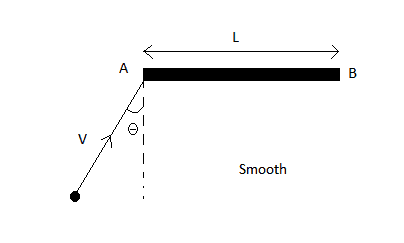## The Attempt at a Solution

T[/B]he image was provided with the question.

When two things collide equal and opposite impulses acts along the direction perpendicular to the contact surface. So in this situation if the particle collides with rod at point A then the impulse which acts on the rod passes through the CoM of the rod. So no impulsive torque acts on the rod about its CoM. So the rod will not rotate. And without rotating, it is not possible for the particle to collide again at point B.So, how this can be solved?

Satvik Pandey said:
. So in this situation if the particle collides with rod at point A then the impulse which acts on the rod passes through the CoM of the rod.
You might want to rethink this. Do you understand force vectors?

Satvik Pandey said:

## The Attempt at a Solution

T[/B]he image was provided with the question.

When two things collide equal and opposite impulses acts along the direction perpendicular to the contact surface. So in this situation if the particle collides with rod at point A then the impulse which acts on the rod passes through the CoM of the rod.

No, why do you think so? The particle collides with the side of the rod near the end, not with the cross-section.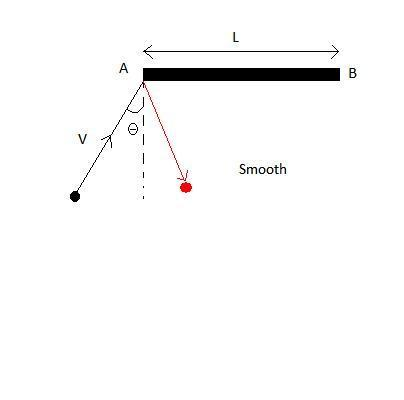•Satvik Pandey
ehild said:
No, why do you think so? The particle collides with the side of the rod near the end, not with the cross-section.

View attachment 77677

OK. But it is given that we have to assume that the particle is point size and the rod is thin. So if that particle collide at the ##edge## we have to assume that edge to be point (because rod is very thin). So how common normal direction can be drawn perpendicular to the contact surface. So I have assume that it collides little bit to the right of the edge.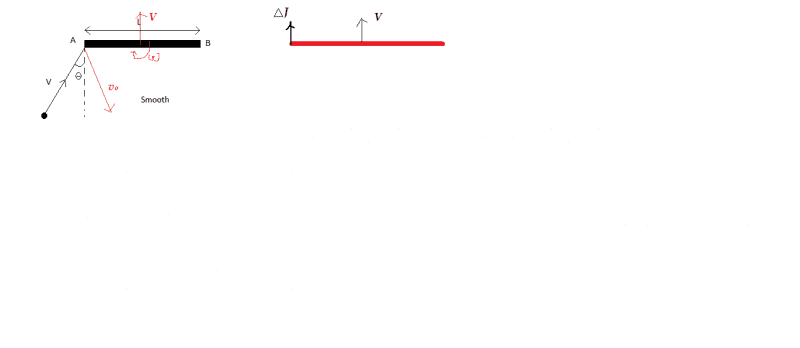I think velocity of the CoM of the rod will be in vertical direction because the impulse acts in vertical direction only.
As the collision is elastic so ##e=1##.
So we can equate the Velocity of approach and velocity of separation in common normal direction.

##V_{app}=v cos\theta## and ##V_{sep}=V+\frac{l\omega}{2}+v_{0} cos\alpha## (##\alpha## is the angle between ##v_{0}## and the common normal direction.

As no force in common tangent direction so ##v sin\theta=v_{0} sin\alpha##

Impulse acting on the particle is equal ti its change in velocity in the common normal direction. right?

So ##J=\triangle V##

So ##J=-m_{1}(v_{0}cos\alpha+vcos\theta)## (Considering downward direction as -ve).

The same impulse acts on the rod.

##{ \tau }_{ imp }=\frac{l}{2}J## (##{ \tau }_{ imp }## is the impulsive torque)

This impulsive torque is equal to the change in the angular momentum of the rod. right?

So ##\frac{l}{2}J=L_{f}##

or ##\frac{l}{2}J=I\omega##

Is the impulse due to collision is equal to the change in the momentum of the CoM of the rod?

If yes then ##J=MV##

Am I right till here?

Satvik Pandey said:
I think velocity of the CoM of the rod will be in vertical direction because the impulse acts in vertical direction only
Really?

phinds said:
Really?

If that particle collide at the edge we have to assume that edge to be point (because rod is very thin). So how common normal direction can be drawn perpendicular to the contact surface. So I have assume that it collides little bit to the right of the edge. Is it fine to do so?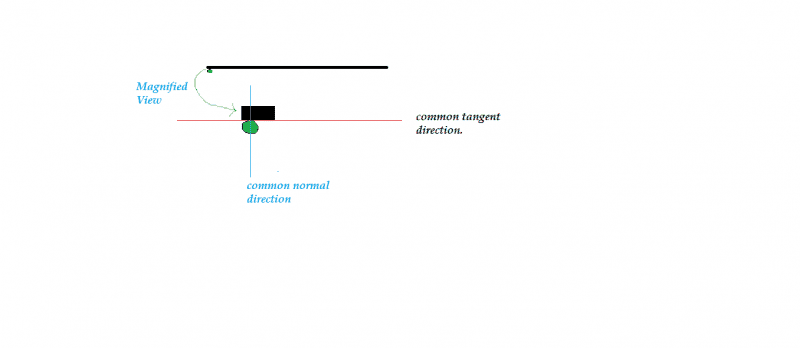During collision equal and opposite impulse acts in the common normal direction. So impulse acts in the vertical direction.

You are treating the point of collision as though it was not part of the rod. No, it is not fine to do so.

phinds said:
You are treating the point of collision as though it was not part of the rod. No, it is not fine to do so.

I don't get you.In the diagram I have shown that the particle collides with the rod so the point of collision lies on the rod.

Satvik Pandey said:
I don't get you.In the diagram I have shown that the particle collides with the rod so the point of collision lies on the rod.
Yeah, I expressed that badly. What I'm trying to point out to you is that you have to treat the rod as a macro object. You hit the rod at an angle and then you are totally ignoring the angle by somehow deciding that it doesn't matter and that you can just throw away the horizontal component of the strike force. That's why I asked you in post #2 if you understand force vectors.

phinds said:
You hit the rod at an angle and then you are totally ignoring the angle by somehow deciding that it doesn't matter and that you can just throw away the horizontal component of the strike force.

So are trying to say that I am neglecting the horizontal component of impulse. I have a confusion. During collision equal and opposite impulse acts in common normal direction.right? Isn't this true for macro object?

Assuming the collision instantaneous, the tangential force (friction) between the rod and particle can be ignored.
The rod can be considered very thin, practically one-dimensional, like a line segment, and the particle arrives at the line segment very near of the end, and rebounds from it. You can take the force of interaction perpendicular to the line representing the rod. The tangential component of momentum of the particle does not change during the collision. The normal components of the momenta do change, both that of the particle and the rod while the total momentum of the system is conserved.
The angular momentum of the whole system is also conserved. The particle has some angular momentum with respect to the CoM of the rod. It will be different after the collision, and the rod gains some angular momentum.

Last edited:
•Satvik Pandey
ehild said:
Assuming the collision instantaneous, the tangential force (friction) between the rod and particle can be ignored.
The rod can be considered very thin, practically one-dimensional, like a line segment, and the particle arrives at the line segment very near of the end, and rebounds from it. You can take the force of interaction perpendicular to the line representing the rod. The tangential component of momentum of the particle does not change during the collision. The normal components of the momenta do change, both that of the particle and the rod while the total momentum of the system is conserved.
The angular momentum of the whole system is also conserved. The particle has some angular momentum with respect to the CoM of the rod. It will be different after the collision, and the rod gains some angular momentum.

Thank you ehild for the explanation.Are we neglecting tangential force (friction) between the rod and particle because the surfaces are friction less or due to instantaneous collision? If the surfaces were not friction less then we have to consider horizontal impulse due to friction. right?

What do you think about my calculations in #post4.

Last edited:
ehil [QUOTE="Satvik Pandey said:
So are trying to say that I am neglecting the horizontal component of impulse. I have a confusion. During collision equal and opposite impulse acts in common normal direction.right? Isn't this true for macro object?
Sorry. I screwed up. Ehild has given a good response.

Satvik Pandey said:
Thank you ehild for the explanation.Are we neglecting tangential force (friction) between the rod and particle because the surfaces are friction less or due to instantaneous collision? If the surfaces were not friction less then we have to consider horizontal impulse due to friction. right?

What do you think about my calculations in #post4.

Edit: Wrong statement: In case of instantaneous collision, you can ignore friction even then when it exists.
Check your calculations, I can not follow them. Explain the notations. You seem to ignore the masses.

Last edited:
Satvik Pandey said:
OK. But it is given that we have to assume that the particle is point size and the rod is thin. So if that particle collide at the ##edge## we have to assume that edge to be point (because rod is very thin). So how common normal direction can be drawn perpendicular to the contact surface. So I have assume that it collides little bit to the right of the edge.

I think velocity of the CoM of the rod will be in vertical direction because the impulse acts in vertical direction only.
As the collision is elastic so ##e=1##.
So we can equate the Velocity of approach and velocity of separation in common normal direction.

##V_{app}=v cos\theta## and ##V_{sep}=V+\frac{l\omega}{2}+v_{0} cos\alpha## (##\alpha## is the angle between ##v_{0}## and the common normal direction.

The velocity of separation is meaningless here. You can not treat the road as a point where the collision occurs.
You have two write out conservation of energy taking both translational and rotational energies into account.

Satvik Pandey said:
As no force in common tangent direction so ##v sin\theta=v_{0} sin\alpha##

Impulse acting on the particle is equal ti its change in velocity in the common normal direction. right?

So ##J=\triangle V##

Do not forget the mass.

Satvik Pandey said:
So ##J=-m_{1}(v_{0}cos\alpha+vcos\theta)## (Considering downward direction as -ve).

The same impulse acts on the rod.

##{ \tau }_{ imp }=\frac{l}{2}J## (##{ \tau }_{ imp }## is the impulsive torque)

This impulsive torque is equal to the change in the angular momentum of the rod. right?

So ##\frac{l}{2}J=L_{f}##

or ##\frac{l}{2}J=I\omega##

Is the impulse due to collision is equal to the change in the momentum of the CoM of the rod?

If yes then ##J=MV##

Am I right till here?

It looks correct , but I would use conservation of momentum and angular momentum instead of the impulse-change of momentum relations. And you miss the equation for conservation of energy.

ehild said:
In case of instantaneous collision, you can ignore friction even then when it exists.
It's not relevant to this question, but that statement is not correct.
In an oblique impact, the normal impulse being J, the tangential impulse is up to ##J \mu_s##. What happens past that is an interesting question. Does ##\mu_k## come into play?

haruspex said:
It's not relevant to this question, but that statement is not correct.
In an oblique impact, the normal impulse being J, the tangential impulse is up to ##J \mu_s##. What happens past that is an interesting question. Does ##\mu_k## come into play?
You are right, as always.:) I think the tangential force would be impulsive, too, and would change the tangential velocities.
Fortunately, the problem says that the collision is elastic, so there is no friction.

ehild said:
The velocity of separation is meaningless here. You can not treat the road as a point where the collision occurs.

I have a doubt regarding the validity of the coefficient of restitution. I have equated the velocity of approach and separation of the particle and the point on which the particle collided with the rod. Can this concept be applied for extended bodies which might involve a combination of both rotation and translation ?ehild said:
Do not forget the mass.

Sorry. That was a typo.:s

ehild said:
It looks correct , but I would use conservation of momentum and angular momentum instead of the impulse-change of momentum relations. And you miss the equation for conservation of energy.

By conservation of energy I got

##\frac { 1 }{ 2 } m{ v }^{ 2 }=\frac { 1 }{ 2 } M{ V }^{ 2 }+\frac { 1 }{ 2 } I{ \omega }^{ 2 }+\frac { 1 }{ 2 } m{ v }_{ 0 }^{ 2 }##

By conserving angular momentum about the CoM of the rod I got

##\frac { mvlcos(\theta ) }{ 2 } =-\frac { m{ v }_{ 0 }lcos\alpha }{ 2 } +I\omega ##

Considering 'clock' as -ve.

Here ##m## and ##M## are the masses of the particle and rod respectively.

What is the condition necessary for the particle to collide again at point B? I know that point B and particle must be at same place at a given time. But I don't know how to frame this constraint equation.

phinds said:
Sorry. I screwed up. Ehild has given a good response.

It's OK.:);)

Satvik Pandey said:
I have a doubt regarding the validity of the coefficient of restitution. I have equated the velocity of approach and separation of the particle and the point on which the particle collided with the rod. Can this concept be applied for extended bodies which might involve a combination of both rotation and translation ?Yes, that concept applies to this problem. It can take the place of the equation for conservation of energy.

By conservation of energy I got

##\frac { 1 }{ 2 } m{ v }^{ 2 }=\frac { 1 }{ 2 } M{ V }^{ 2 }+\frac { 1 }{ 2 } I{ \omega }^{ 2 }+\frac { 1 }{ 2 } m{ v }_{ 0 }^{ 2 }##
OK

By conserving angular momentum about the CoM of the rod I got

##\frac { mvlcos(\theta ) }{ 2 } =-\frac { m{ v }_{ 0 }lcos\alpha }{ 2 } +I\omega ##

Considering 'clock' as -ve.

The signs can be a headache. Are you taking ##\omega## to be a negative quantity if it is clockwise? Anyway, double check that you have the signs correct. Also, keep in mind that the particle need not "bounce back". It might retain an "upward" component of velocity after the collision.

What is the condition necessary for the particle to collide again at point B? I know that point B and particle must be at same place at a given time. But I don't know how to frame this constraint equation.

It might be good to consider things from the reference frame of the CM of the rod after the collision.

TSny said:
Yes, that concept applies to this problem. It can take the place of the equation for conservation of energy.

I use this concept and I got ##v cos\theta=V+\frac{l\omega}{2}+v_{0} cos\alpha##. I mentioned that in #post4. Is this correct?

TSny said:
The signs can be a headache. Are you taking ##\omega## to be a negative quantity if it is clockwise? Anyway, double check that you have the signs correct. Also, keep in mind that the particle need not "bounce back". It might retain an "upward" component of velocity after the collision.

I have taken clockwise direction as +ve. I just wrote that opposite..But this equation satisfy the two equation which I got from using impulse and momentum relation. ##MV=m_{1}(v_{0}cos\alpha+vcos\theta)## and ##\frac{l}{2}MV=I\omega##.

Satvik Pandey said:
I use this concept and I got ##v cos\theta=V+\frac{l\omega}{2}+v_{0} cos\alpha##. I mentioned that in #post4. Is this correct?

Yes, that looks correct. You are defining ##v_{0} cos\alpha## to be be the downward component of the final velocity of the particle; whereas, ##v cos\theta## is the upward component of the initial velocity of the particle.

I have taken clockwise direction as +ve. I just wrote that opposite..But this equation satisfy the two equation which I got from using impulse and momentum relation. ##MV=m_{1}(v_{0}cos\alpha+vcos\theta)## and ##\frac{l}{2}MV=I\omega##.

OK. I think it's good.

TSny said:
Yes, that looks correct. You are defining ##v_{0} cos\alpha## to be be the downward component of the final velocity of the particle; whereas, ##v cos\theta## is the upward component of the initial velocity of the particle.

Yes I have considered ##v_{0} cos\alpha## to be be the downward component of the final velocity of the particle; whereas, ##v cos\theta## is the upward component of the initial velocity of the particle.

How to get that constraint equation?Satvik Pandey said:
How to get that constraint equation?After the collision, what do the trajectories of the particle and point B of the rod look like in the frame of reference of the CM of the rod?

•Satvik Pandey
TSny said:
After the collision, what do the trajectories of the particle and point B of the rod look like in the frame of reference of the CM of the rod?

I got velocity of particle (let it be M) wrt CoM is ##{ v }_{ 0 }sin\alpha \hat { i } -{ (v }_{ 0 }cos\alpha +V)\hat { j }##

So ##tan\phi =\frac { { (v }_{ 0 }cos\alpha +V) }{ { v }_{ 0 }sin\alpha } ##.
Where ##\phi## is the angle between velocity of the particle and Y axis.

And the point B moves in a circle around with radius ##\frac{l}{2}##.

Should I use calculus to find relative velocity at any time 't' and then find its projection along the distance between M and B at that time and then express this velocity as dx/dt and then integrate within proper limits. But I think that equation will contain lots of extra variable.

Last edited:
Satvik Pandey said:
I got velocity of particle (let it be M) wrt CoM is ##{ v }_{ 0 }sin\alpha \hat { i } -{ (v }_{ 0 }cos\alpha +V)\hat { j }##

So ##tan\phi =\frac { { (v }_{ 0 }cos\alpha +V) }{ { v }_{ 0 }sin\alpha } ##.
Where ##\phi## is the angle between velocity of the particle and Y axis.

Did you mean X axis here?

And the point B moves in a circle around with radius ##\frac{l}{2}##.

Should I use calculus to find relative velocity at any time 't' and then find its projection along the distance between M and B at that time and then express this velocity as dx/dt and then integrate within proper limits. But I think that equation will contain lots of extra variable.
That does sound messy.

I would think about where the particle crosses the circular trajectory of B.

[Is there supposed to be an explicit expression for the solution to this problem? I'm getting a transcendental equation for θ that I can only solve numerically. Also, I get solutions only for a certain range of the ratio of the two masses.]

•Satvik Pandey
TSny said:
[Is there supposed to be an explicit expression for the solution to this problem? I'm getting a transcendental equation for θ that I can only solve numerically. Also, I get solutions only for a certain range of the ratio of the two masses.]
I found the same. Rod needs to have more than 4 times the mass of the projectile, right?

I'm getting that the ratio of rod to particle masses must lie between approximately 0.7 and 8. But I could certainly be wrong here. If the mass of the rod is greater than 4 times the mass of the particle, then the particle "bounces back" in the original reference frame. Otherwise, the particle continues in an upward motion after the collision in this frame.

TSny said:
I'm getting that the ratio of rod to particle masses must lie between approximately 0.7 and 8. But I could certainly be wrong here. If the mass of the rod is greater than 4 times the mass of the particle, then the particle "bounces back" in the original reference frame. Otherwise, the particle continues in an upward motion after the collision in this frame.

Satvik Pandey said:
I got velocity of particle (let it be M) wrt CoM is ##{ v }_{ 0 }sin\alpha \hat { i } -{ (v }_{ 0 }cos\alpha +V)\hat { j }##

So ##tan\phi =\frac { { (v }_{ 0 }cos\alpha +V) }{ { v }_{ 0 }sin\alpha } ##.
Where ##\phi## is the angle between velocity of the particle and Y axis.

And the point B moves in a circle around with radius ##\frac{l}{2}##.

Should I use calculus to find relative velocity at any time 't' and then find its projection along the distance between M and B at that time and then express this velocity as dx/dt and then integrate within proper limits. But I think that equation will contain lots of extra variable.

First find the expressions for the unknown vo, α, V, and ω in terms of the known data.
The particle moves along a straight line, with constant velocity, starting from (-L/2, 0). B moves around the origin, along a circle of radius L/2. The line intersects the circle. Where? From the point of intersection, you can find theta in principle.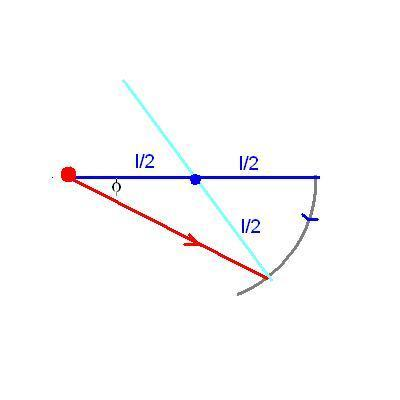Last edited:
•Satvik Pandey
I got ##V=\frac { 2mvcos\theta }{ 4m+M\\ } \quad ##. Using equation ##v cos\theta=V+\frac{l\omega}{2}+v_{0} cos\alpha## and

##6V=l\omega## and ##MV=m(v_{0}cos\alpha+vcos\theta)##

and I got ##\omega =\frac { 6 }{ l } (\frac { 2mvcos\theta }{ 4m+M } )##

and ##\alpha =arcsin(\frac { vsin\theta }{ { v }_{ 0 } } )##

So ##cos\alpha =\frac { \sqrt { { v }_{ 0 }-{ v }^{ 2 }{ sin }^{ 2 }\theta } }{ { v }_{ 0 } } ##

And from energy conservation equation I got

##{ v }_{ 0 }=\sqrt { { v }^{ 2 }-\frac { 4M{ V }^{ 2 } }{ m } } ##

or ##{ v }_{ 0 }=\sqrt { { v }^{ 2 }-\frac { 4M }{ m } { \left( \frac { 2mvcos\theta }{ 4m+M\\ } \right) }^{ 2 } } ##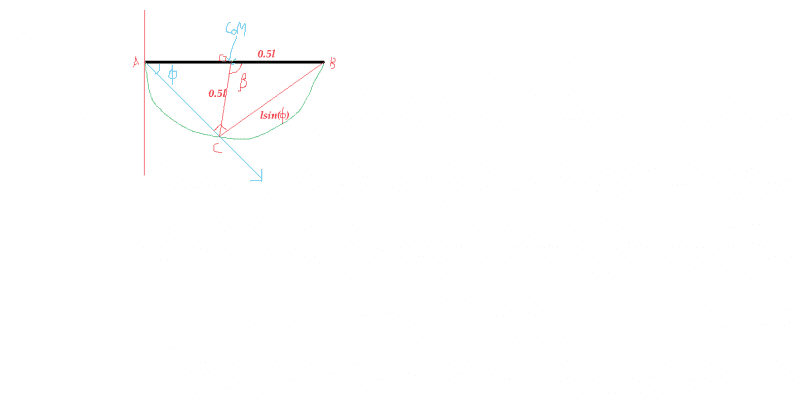Now angle ACB is 90. So ##BC=lsin\phi##

Now bu applying cosine rule in triangle OBC I got

##{ (lsin\phi ) }^{ 2 }=\left( { \frac { l }{ 2 } } \right) ^{ 2 }+\left( { \frac { l }{ 2 } } \right) ^{ 2 }-2\frac { { l }^{ 2 } }{ 4 } cos(\beta )##

I have an expression of ##\phi## in terms of ##v_{0}## ##V## and ##\alpha##. Am I right till here?

TSny said:
Is there supposed to be an explicit expression for the solution to this problem? I'm getting a transcendental equation for θ that I can only solve numerically. Also, I get solutions only for a certain range of the ratio of the two masses.]

I forgot to mention an information in the question.It is given that ##\frac { M }{ m } =\frac { 12 }{ \pi } +2##.
Here ##M## and ##m## are the masses of rod and the particle respectively.

This might help.

Satvik Pandey said:
I forgot to mention an information in the question.It is given that ##\frac { M }{ m } =\frac { 12 }{ \pi } +2##.
Here ##M## and ##m## are the masses of rod and the particle respectively.

This might help.

It does! Why did not say earlier ?

Have you noticed that β=2Φ?

•Satvik Pandey
ehild said:
It does! Why did not say earlier ?

Have you noticed that β=2Φ?

Yeah! β=2Φ. I think I should put it in last equation in #post31. I think I now have sufficient number of equation to find ##\theta##. right?. Are my calculations in post #31 correct?

Satvik Pandey said:
Yeah! β=2Φ. I think I should put it in last equation in #post31. I think I now have sufficient number of equation to find ##\theta##. right?. Are my calculations in post #31 correct?

No, it will give an identity. Use that the time for the particle to go from A to C is the same as β/ω. You know the speed of the particle in terms of known data.
Let us see what you get.

•Satvik Pandey